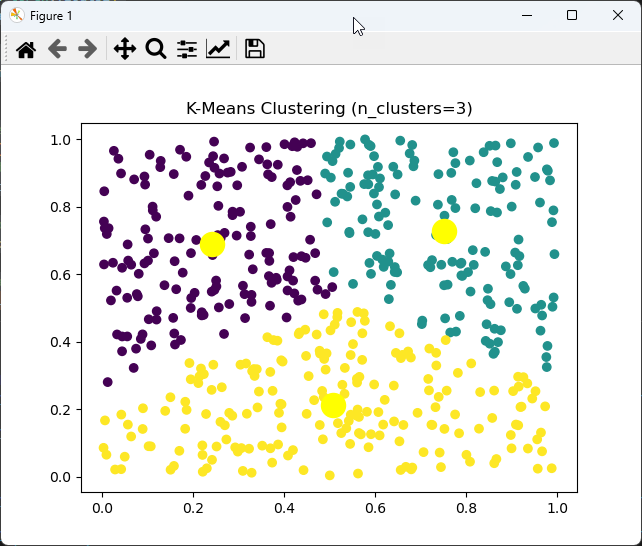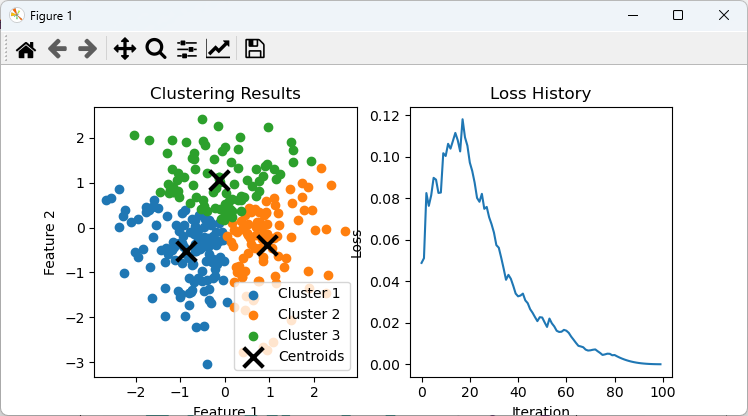Python

Cluster - pyTorch

In this note, I want to try to apply an approach that is completely from other notes. I wanted to use chatGPT to create a Python code that I want instead of writing it myself.

NOTE : Refer to this note for my personal experience with chatGPT coding and advtantage & limitation of the tool. In general, I got very positive impression with chatGPT utilization for coding.

This code is created first by chatGPT on Feb 03 2023 (meaning using chatGPT 3.5). For this code, I haven't started from the requirement. I asked chatGPT to write the same function as this using pyTorch.

As you notice, this code uses some math function of torch, but does not use any neural net.

NOTE : To my surprise (in negative way), chatGPT coding was a little bit disappointing in terms of pyTorch coding. It seems chatGPT became dumb. In most case, the initial response was the code with syntax errors and when I said 'fix it' it rewrite the entire code in complete different way instead of the fixing a specific part and the new code generates other syntax error. At first, I thought chatGPT would write stable, high quality Python code because I assumed that it has been trained most extensively for Python because I thought there are the most amount of python code shared by gitHub or stackoverflow etc.

 Cluster_kCluster_PyTorch_01.py import torch import matplotlib.pyplot as plt   def kmeans(data, n_clusters, n_init=10, max_iter=300, tol=0.0001, random_state=0):       data = torch.tensor(data, dtype=torch.float32)     num_points, num_dims = data.shape       # initialize cluster centroids randomly     centroids = data[torch.randperm(num_points)[:n_clusters]]       for i in range(max_iter):         # calculate distances between each point and the centroids         distances = torch.stack([((data - centroids[j])**2).sum(dim=1) for j in range(n_clusters)])           # find the closest centroid for each data point         closest_centroids = distances.argmin(dim=0)           # update the centroids to be the mean of the data points assigned to them         new_centroids = torch.stack([data[closest_centroids == j].mean(dim=0) for j in range(n_clusters)])           # check if the centroids have changed         if (new_centroids - centroids).abs().max() < tol:             break         centroids = new_centroids       return closest_centroids, centroids   def display_result(X, y_pred, n_clusters, centroids):     X = torch.tensor(X, dtype=torch.float32)     plt.scatter(X[:, 0], X[:, 1], c=y_pred)     plt.scatter(centroids[:, 0], centroids[:, 1], s=300, c='yellow', label='Centroids')     plt.title(f'K-Means Clustering (n_clusters={n_clusters})')     plt.show()   def test_kmeans():     # Generate random sample data with 100 samples and 2 features     n_samples = 500     n_features = 2     X = torch.rand(n_samples, n_features).numpy()     n_clusters = 3       # Call the kmeans function     labels, centroids = kmeans(X, n_clusters)       # Assert that the shape of the labels is correct     assert labels.shape == (n_samples,), f"Expected shape {(n_samples,)}, but got {labels.shape}"       # Assert that the shape of the centroids is correct     assert centroids.shape == (n_clusters, n_features), f"Expected shape {(n_clusters, n_features)}, but got {centroids.shape}"       # Display the result     display_result(X, labels, n_clusters, centroids)   test_kmeans()

The result from this code is as follows :I asked chatGPT to use 'feedforward neural net' and got the same code and plot clustered output and loss values in the same figure.

NOTE : I expected a little bit different code from this. I expected chatGPT to use multiple layers of linear layers but the result is not what I expected. Probably the term 'feedforward' in my request was interpreted differently. But the outcome of the execution is exactly what I wanted get.

 Cluster_kCluster_PyTorch_NN_01.py import torch import torch.nn as nn import torch.optim as optim import numpy as np import matplotlib.pyplot as plt   def k_means_clustering_nn(X, n_clusters, n_iter=100):     X = torch.tensor(X, dtype=torch.float32)     n_samples, n_features = X.shape     centroids = torch.randn((n_clusters, n_features), dtype=torch.float32)     cluster_assignment = torch.zeros((n_samples,), dtype=torch.int64)     loss_hist = []       class KMeansClusteringNN(nn.Module):         def __init__(self):             super(KMeansClusteringNN, self).__init__()             self.centroids = nn.Parameter(torch.randn((n_clusters, n_features), dtype=torch.float32))              def forward(self, X):             X = X.unsqueeze(1)             distances = (X - self.centroids)**2             distances = torch.sum(distances, dim=2)             cluster_assignment = torch.argmin(distances, dim=1)             return cluster_assignment       model = KMeansClusteringNN()     optimizer = optim.Adam(model.parameters(), lr=1e-2)     for i in range(n_iter):         optimizer.zero_grad()         cluster_assignment = model(X)         loss = 0         for c in range(n_clusters):             points_in_cluster = X[cluster_assignment == c]             mean_of_points = torch.mean(points_in_cluster, dim=0)             centroids_loss = torch.sum((mean_of_points - model.centroids[c])**2)             loss += centroids_loss         loss /= n_clusters         loss.backward()         optimizer.step()         loss_hist.append(loss.item())          with torch.no_grad():         final_cluster_assignment = model(X)         final_centroids = model.centroids.detach().numpy()          return final_centroids, final_cluster_assignment.numpy(), loss_hist   # generate sample data np.random.seed(0) X = np.random.randn(300, 2) n_clusters = 3 centroids, cluster_assignment, loss_hist = k_means_clustering_nn(X, n_clusters)     fig, ax = plt.subplots(1, 2, figsize=(12, 4))   # plot clustering results for c in range(n_clusters):     points_in_cluster = X[cluster_assignment == c]     ax.scatter(points_in_cluster[:, 0], points_in_cluster[:, 1], label=f'Cluster {c+1}') ax.scatter(centroids[:, 0], centroids[:, 1], marker='x', color='k', s=200, linewidth=3, label='Centroids') ax.set_xlabel('Feature 1') ax.set_ylabel('Feature 2') ax.set_title('Clustering Results') ax.legend()   # plot loss history ax.plot(loss_hist) ax.set_xlabel('Iteration') ax.set_ylabel('Loss') ax.set_title('Loss History')   plt.show()

The result from this code is as follows :